## IYZ Problem Solving and Programming | Problem Solving Portfolio Task 3

Weight = 35%, Total marks = 30

1.            Write a program, using array data structures, that presents the days of the week and the highest temperatures on each of those days of the week.  Include functions to calculate the average highest temperature and the minimum and the maximum temperatures over the period of days.

(6 marks)

2.            Explain how the Insertion Sort algorithm works. Give a pseudo code algorithm. Illustrate your answer graphically by showing the operation of the algorithm applied to the following data:

95, 72, 21, 48

3.            a.     Explain why computers can only process data in binary form.

b.       Identify the situations where it would be appropriate to use the following units of measurement. Provide examples in each case

i.             Kilobyte

ii.             Megabyte

iii.             Terabyte

(4 marks)

4.             Perform the following number system conversions:

b.           Convert 110010112 to decimal notation

c.            Convert 3FA716 to decimal notation

d.           Convert F2A7 to binary notation

e.           Convert 218 to binary notation

(8 marks)

5.               Write a program that attempts to remove an item from an array. Your code searches the array a for the item x. if x is found, its first occurrence is removed, all the elements above the position are shifted down and true is returned to indicate a successful removal. If x is not found, the array is left unchanged and false is returned.

(7 marks)

import java.util.Scanner;

public class Solution {
public static void main(String[] args) {
Scanner input = new Scanner(System.in);
int[] highestTempOfDay = new int;
System.out.println("Enter highest temperatures on each of those days of the week:");
// Taking highest tempreture of the day of the week
for(int i=0; i<7; ++i) {
highestTempOfDay[i] = input.nextInt();
}

System.out.println("Average Highest Temp is: "+ averageHighestTemperature(highestTempOfDay));
System.out.println("Minimum Temp of the week is: "+ minTempOfTheWeek(highestTempOfDay));
System.out.println("Maximum Temp of the week is: "+ maxTempOfTheWeek(highestTempOfDay));
input.close();
}

// This method will return average highest tempreture of the week
public static int averageHighestTemperature(int[] highestTempOfDay) {
int totalTemp = 0;
for (int i = 0; i < 7; ++i) {
totalTemp += highestTempOfDay[i];
}
}

// This method return minimum highest tempreture of the week
public static int minTempOfTheWeek (int[] highestTempOfDay) {
int minTempOfTheWeek = highestTempOfDay;
for (int i = 1; i < 7; ++i) {
minTempOfTheWeek = Math.min(minTempOfTheWeek, highestTempOfDay[i]);
}
return minTempOfTheWeek;
}

// This method return maximum highest tempreture of the week
public static int maxTempOfTheWeek(int[] highestTempOfDay) {
int maxTempOfTheWeek = highestTempOfDay;
for (int i = 1; i < 7; ++i) {
maxTempOfTheWeek = Math.max(maxTempOfTheWeek, highestTempOfDay[i]);
}
return maxTempOfTheWeek;
}
}

___________________________________________________________________________

Input:
22 23 21 25 22 27 26

Output:
Average Highest Temp is: 23
Minimum Temp of the week is: 21
Maximum Temp of the week is: 27

Working of Insertion – Sort algorithm :
In insertion - sort sorted algorithm elements are sorted sequentially wise. First from index 0 to 1 are being sorted then 0 to 2 then 0 to 3 and so on till index n – 1. i.e. In this algorithm we peek one element at a time and insert it into its correct position by comparing from the element which is right side form this element.

Pseude Code of Insertion Sort Algorithm

Insertion-Sort(A)
{
For j = 2 to A.length
Key = A[j]
// Insert A[j] into sorted sequence A[1…j-1]
i = j – 1
While( i > 0 and A[i] > key)
A[i + 1] = A[i]
i = i – 1
A[i + 1] = key

}

Graphically Representation:

Steps:
Starting Insertion Sort
The left side of green record will be sorted always and we will begin with the record at position 0 and move till record at position 1 which is blue until the whole array will be sorted.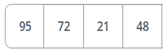Steps:

Swap.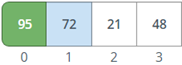Steps:

Move the blue record to the left until it reaches the correct position.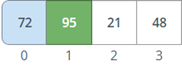Steps:

Processing record in position 3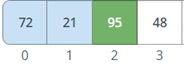Steps:

Move the blue record to the left until it reaches the correct position.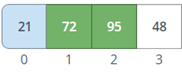Steps:

Swap.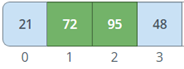Steps:

Processing record in position 4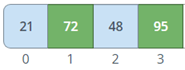Steps:

Move the blue record to the left until it reaches the correct position.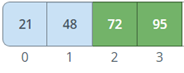Steps:

Done sorting!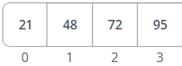a.    Every number in computer is an electrical signal, which can be either on or off. So, only two state are possible for an electrical signal , Therefore computers can only process data in binary form.

b.
i.  Kilobyte :  One kilobyte is equal to 1024 byte. Kilobyte is abbreviated as ‘KB’. It is mostly used measure small file size, such as text document.

ii. Megabyte : One Megabyte is equal to 1024 ‘KB’.  Megabyte is abbreviated as ‘MB’. It is mostly used to measure medium file size, such as a high resolution JPEG image.

iii. Terabyte : One Terabyte is equal to 1 trillion bytes. Terabyte is abbreviated as ‘TB’.   It contains exactly 1, 099, 511, 627, 776 bytes or 1, 024 GB. It is mostly used to measure big file size such as storage of server or data center.

4.

a.  11001011  =   (1 * 2^7)  + (1 * 2^6)  + (0 * 2^5)  + (0* 2^4)  + (1 * 2^3)  + (0* 2^2)  + (1 * 2^1)  + (1 * 2^0)

=  128 + 64  + 0 +  0  + 8 +  0  + 2  + 1  =  203

b.  3FA7       =  (3  * 16^3 )   +   (15 * 16^ 2)   +   (10  * 16^ 1)   + (7  * 16^0 )

=  3 *  4096  +  15 * 256   + 10 * 16  + 7 * 1   =  16295

c.   F2A7     =   1111001010100111

d.   218       =    11011010

5.

public static boolean removeFirstOccurance(int[] aint x) {

boolean isItemFound = false;

int i = 0;

// Searching for Item

for(; i < a.length; ++i) {

if(a[i] == x) {

isItemFound = true;

break;

}

}

// Shifting 1 position if Item found

if(isItemFound) {

for(int j = i+1j < a.length; ++j) {

a[j-1] = a[j];

}

}

return isItemFound;

}

Share: